# Math in Focus Grade 3 Chapter 6 Practice 5 Answer Key Multiply by 9

Go through the Math in Focus Grade 3 Workbook Answer Key Chapter 6 Practice 5 Multiply by 9 to finish your assignments.

## Math in Focus Grade 3 Chapter 6 Practice 5 Answer Key Multiply by 9

Complete the multiplication fact. Then show on the number line.

Question 1.
2 × 9 = ___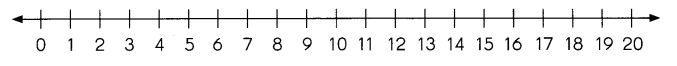2 × 9 = 18.

Explanation:
Here, for the multiplication 2 × 9, there will be 2 jumps of 9. So the number line will be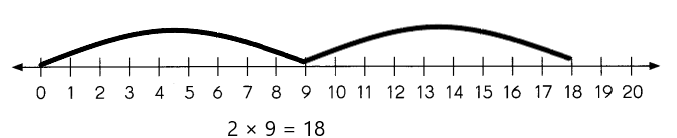Complete the multiplication fact. Then show on the area model.

Question 2.
7 × 9 = ___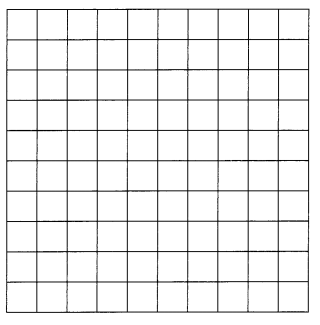7 × 9 = 63.

Explanation:
The multiplication of 7 × 9 is 63. So the model of 7 × 9 is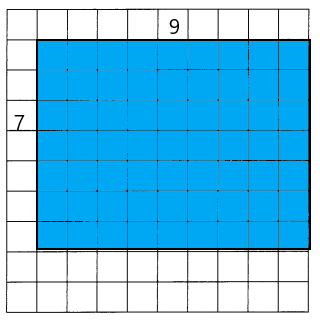Fill in the missing numbers.

Question 3.
3 nines = 3 × ___
3 nines = 3 × 9.

Explanation:
Given that 3 nines which is 3 × 9 = 27. So 3 nines is 3 × 9 = 27.

Question 4.
4 nines = ___ × 9
4 nines = 4 × 9.

Explanation:
Given that 4 nines which is 4 × 9 = 36. So 4 nines is 4 × 9 = 36.

Fill in the missing numbers.

Question 5.
9 + 9 + 9 + 9 + 9 + 9 = 6 × _____
9 + 9 + 9 + 9 + 9 + 9 = 6 × 9

Explanation:
Given that 9 + 9 + 9 + 9 + 9 + 9 which is 6 × 9 = 54.

Question 6.
6 + 6 + 6 + 6 + 6 + 6 + 6 + 6 + 6 = 9 × _____
6 + 6 + 6 + 6 + 6 + 6 + 6 + 6 + 6 = 9 × 6

Explanation:
Given that 6 + 6 + 6 + 6 + 6 + 6 + 6 + 6 + 6 which is 9 × 6 = 54.

Question 7.
9 × 8 = 8 × ______
9 × 8 = 8 × 9.

Explanation:
Given that 9 × 8 so it will be 8 × 9 which is 72.

Question 8.
8 nines = 9 + 9 + 9 + 9 + 9 + 9 + ______ + ______
8 nines = 9+9+9+9+9+9+9+9+9.

Explanation:
Given that 8 nines which is 9+9+9+9+9+9+9+9+9 = 72.

Question 9.
10 × 4 = ____
9 × 4 = 10 groups of 4 – __ groups of 4
= ___ – ____
= _____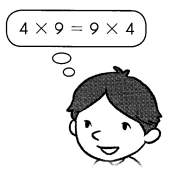9 × 4 = 36.

Explanation:
Given that 10 × 4 is 40 and there are 10 groups of 4, so for 9 × 4 it will be
9 × 4 = 10 groups of 4 – 1 group of 4
= 40 – 4
= 36.

Question 10.
10 × 9 = ___
9 × 9 = 10 groups of 9 – ___ group of 9
= ____ – ____
= ____
10 × 9 = 90
9 × 9 = 81

Explanation:
Given that 10 × 9 is 90 and there are 10 groups of 9, so for 9 × 9 it will be
9 × 9 = 10 groups of 9 – 1 group of 9
= 90 – 9
= 81.

Question 11.
5 × 9 = ___
6 × 9 = 5 groups of 9 + ___ group of 9
= ___ + ___
= ___
5 × 9 = 45,
6 × 9 = 54

Explanation:
Given that 5 × 9 is 45 and there are 5 groups of 9, so for 6× 9 it will be
5 × 9 = 45
6 × 9 = 5 groups of 9 + 1 group of 9
= 45 + 9
= 54.

Match each ball to the correct basket.

Question 12.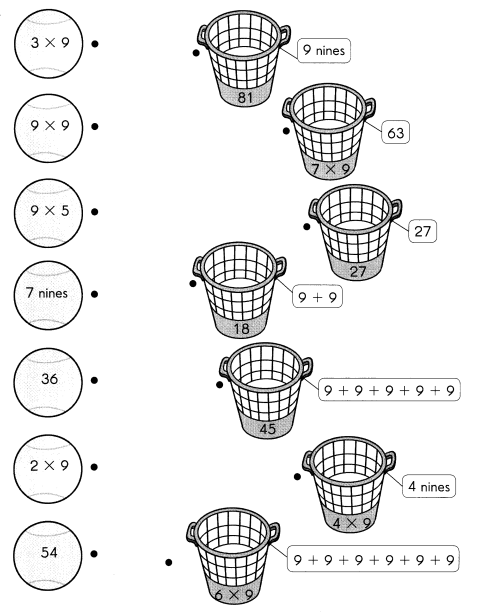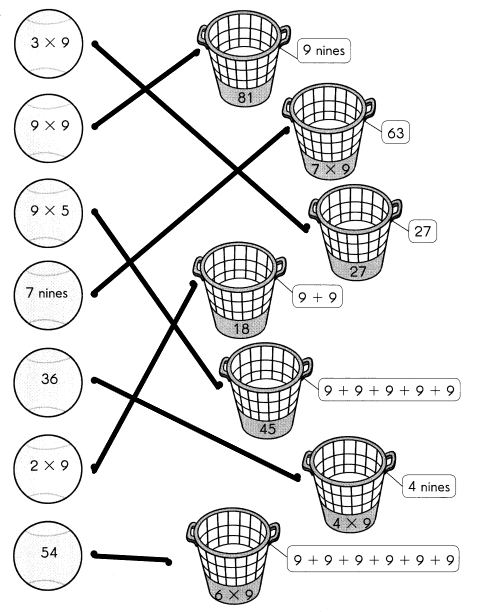Solve.

Question 13.
c How many stickers does she paste in all?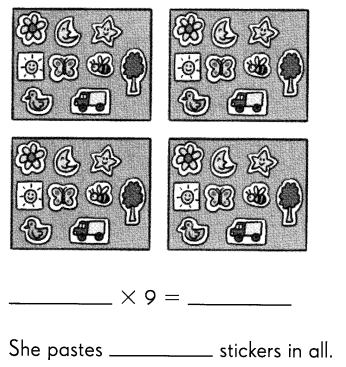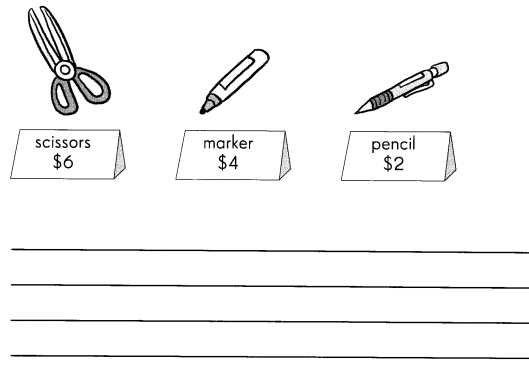Shan bought 4 scissors which cost $6 each, 2 markers which cost$4 each, and 3 pencils which cost $2 each. How much did Shan spend in total? Explanation: Given that Shan bought 4 scissors which cost$6 each which is $6×4 =$24, 2 markers which cost $4 each which is$4×2 = $8, and 3 pencils which cost$2 each which is $2×3 =$6. So the total Shan spend is $24+$8+$6 =$38.